# sfepy.mechanics.membranes module¶

sfepy.mechanics.membranes.create_mapping(coors, gel, order)[source]

Create mapping from transformed (in x-y plane) element faces to reference element faces.

Parameters
coorsarray

The transformed coordinates of element nodes, shape (n_el, n_ep, dim). The function verifies that the all z components are zero.

gelGeometryElement instance

The geometry element corresponding to the faces.

orderint

The polynomial order of the mapping.

Returns
mappingVolumeMapping instance

The reference element face mapping.

sfepy.mechanics.membranes.create_transformation_matrix(coors)[source]

Create a transposed coordinate transformation matrix, that transforms 3D coordinates of element face nodes so that the transformed nodes are in the x-y plane. The rotation is performed w.r.t. the first node of each face.

Parameters
coorsarray

The coordinates of element nodes, shape (n_el, n_ep, dim).

Returns
mtx_tarray

The transposed transformation matrix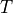, i.e..

Notes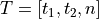, where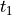,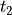, are unit in-plane (column) vectors andis the unit normal vector, all mutually orthonormal.

sfepy.mechanics.membranes.describe_deformation(el_disps, bfg)[source]

Describe deformation of a thin incompressible 2D membrane in 3D space, composed of flat finite element faces.

The coordinate system of each element (face), i.e. the membrane mid-surface, should coincide with the x, y axes of the x-y plane.

Parameters
el_dispsarray

The displacements of element nodes, shape (n_el, n_ep, dim).

bfgarray

The in-plane base function gradients, shape (n_el, n_qp, dim-1, n_ep).

Returns
mtx_c ; array

The in-plane right Cauchy-Green deformation tensor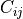,.

c33array

The component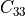computed from the incompressibility condition.

mtx_barray

The discrete Green strain variation operator.

sfepy.mechanics.membranes.describe_geometry(field, region, integral)[source]

Describe membrane geometry in a given region.

Parameters
fieldField instance

The field defining the FE approximation.

regionRegion instance

The surface region to describe.

integralIntegral instance

The integral defining the quadrature points.

Returns
mtx_tarray

The transposed transformation matrix, see create_transformation_matrix().

membrane_geoCMapping instance

The mapping from transformed elements to a reference elements.

sfepy.mechanics.membranes.get_green_strain_sym3d(mtx_c, c33)[source]

Get the 3D Green strain tensor in symmetric storage.

Parameters
mtx_c ; array

The in-plane right Cauchy-Green deformation tensor,, shape (n_el, n_qp, dim-1, dim-1).

c33array

The componentcomputed from the incompressibility condition, shape (n_el, n_qp).

Returns
mtx_earray

The membrane Green strain, symmetric storage: items (11, 22, 33, 12, 13, 23), shape (n_el, n_qp, sym, 1).

sfepy.mechanics.membranes.get_invariants(mtx_c, c33)[source]

Get the first and second invariants of the right Cauchy-Green deformation tensor describing deformation of an incompressible membrane.

Parameters
mtx_c ; array

The in-plane right Cauchy-Green deformation tensor,, shape (n_el, n_qp, dim-1, dim-1).

c33array

The componentcomputed from the incompressibility condition, shape (n_el, n_qp).

Returns
i1array

The first invariant of.

i2array

The second invariant of.

sfepy.mechanics.membranes.get_tangent_stress_matrix(stress, bfg)[source]

Get the tangent stress matrix of a thin incompressible 2D membrane in 3D space, given a stress.

Parameters
stressarray

The components 11, 22, 12 of the second Piola-Kirchhoff stress tensor, shape (n_el, n_qp, 3, 1).

bfgarray

The in-plane base function gradients, shape (n_el, n_qp, dim-1, n_ep).

Returns
mtxarray

The tangent stress matrix, shape (n_el, n_qp, dim*n_ep, dim*n_ep).

sfepy.mechanics.membranes.transform_asm_matrices(out, mtx_t)[source]

Transform matrix assembling contributions to global coordinate system, one node at a time.

Parameters
outarray

The array of matrices, transformed in-place.

mtx_tarray

The transposed transformation matrix, see create_transformation_matrix().

sfepy.mechanics.membranes.transform_asm_vectors(out, mtx_t)[source]

Transform vector assembling contributions to global coordinate system, one node at a time.

Parameters
outarray

The array of vectors, transformed in-place.

mtx_tarray

The transposed transformation matrix, see create_transformation_matrix().